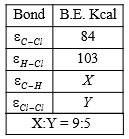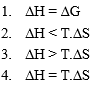The reaction

CH4(g) + Cl2(g)$\to$CH3Cl(g) + HCl(g)

has $∆\mathrm{H}$=-25 K calFrom the given data, what is the bond enthalpy of Cl-Cl bond?

1. 70 K cal                                 2.  80 K cal

3.  67.75 K cal                           4. 57.85 K cal

Concept Questions :-

Enthalpy and It's Type
High Yielding Test Series + Question Bank - NEET 2020

Difficulty Level:

In which case entropy decreases?

1. Boiling of egg

2. Combustion of benzene at 127$°$C

3. Stretching of rubber band

4. Dissolution of sugar in water

Concept Questions :-

Spontaneity and Entropy
High Yielding Test Series + Question Bank - NEET 2020

Difficulty Level:

Which is correct at equilibrium?

1. $∆$H = 0              2. $∆$GTotal=0

3. $∆$STotal=0          4. $∆$E=0

Concept Questions :-

Gibbs Energy Change
High Yielding Test Series + Question Bank - NEET 2020

Difficulty Level:

In which case heat of neutralization is greater than 13.7 K Cal?

1. HF & NH4OH              2. HCl & NaOH

3. HI & KOH                   4. HF & NaOH

Concept Questions :-

Thermochemistry
High Yielding Test Series + Question Bank - NEET 2020

Difficulty Level:

Consider the reaction

N2(g) + 3H2(g)$\to$2NH3(g) carried out at constant temperature and pressure. If $∆$H and $∆$E are enthalpy change and internal energy change respectively, which of the following expression is True?

Concept Questions :-

Enthalpy and It's Type
High Yielding Test Series + Question Bank - NEET 2020

Difficulty Level:

Which work done is maximum?

1. Isobaric work           2. Isothermal work

3. Isochoric work         4. Adiabatic work

Concept Questions :-

Thermodynamics' Properties and process

Difficulty Level:

For exothermic reaction to be spontaneous ($∆\mathrm{S}$=negative)

1. Temperature must be high

2. Temperature must be zero

3. Temperature may have any magnitude

4. Temperature must be low

Concept Questions :-

Spontaneity and Entropy
High Yielding Test Series + Question Bank - NEET 2020

Difficulty Level:

Which of the following conditions should be satisfied for the given reaction to be spontaneous at 0$°$C and 1 atm?

H2O(s) $⇌$H2O(l)Concept Questions :-

Spontaneity and Entropy
High Yielding Test Series + Question Bank - NEET 2020

Difficulty Level:

'The free energy change due to a reaction is zero when

1. The reactants are initially mixed.

3. The system is at equilibrium

4. The reactants are completely consumed

Concept Questions :-

Gibbs Energy Change

Difficulty Level:

An ideal gas expands at a constant external pressure of 2.0 atmosphere by 20 litre and absorbs 10kJ of heat from surrounding. What is the change in internal energy of the system:-

(1) 4052 J

(2) 5948 J

(3) 14052 J

(4) 9940 J

Concept Questions :-

First Law of Thermodynamics